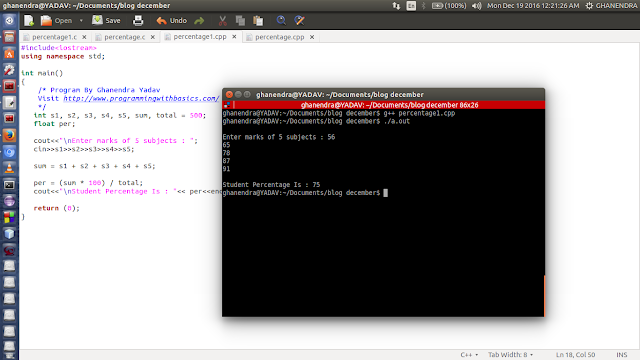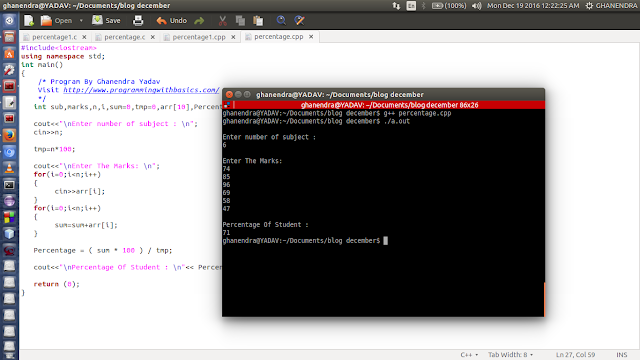# C++ Program to Calculate Percentage of Marks of 6 Subjects

Write a C++ Program to Calculate Percentage of Marks of 6 Subjects. means you have to give a variable size of a subject like 2, 3, 5 and more subjects as the user wants and you need to calculate the percentage of marks and also print the marks of each subject. Program to Calculate percentage in c++.

First, we will ask the user for the total number of subjects after that we will enter the marks of all those subjects. Now we will calculate a percentage of marks and print student marks, percentages, and The percentage formula is given Below.

Percentage Formula in C++

Percentage of Marks = Total Marks / Total Number of Subjects.

How to Calculate Percentage in C++

I am taking 6 subjects as an example. Below are the 5 steps to Calculate the Percentage of Marks.

1. First, ask the user to enter the total number of subjects.
2. Stores the marks of subjects in an array,
3. Add the Marks of Subjects which already been entered by the user.
4. Now apply the Percentage of Marks formula.
5. Print the Percentage of Marks calculated by the formula.

Tip: Always read the full post so you can understand better and check other solutions in different-different languages.

## C++ Program to Calculate Percentage of Marks

Method 1: This method is helpful when the number of subjects is not fixed. The below program can Calculate the Percentage of Marks, Any number of  Subjects. Total Subject could be any number of sizes it may be 2, 3, 4, 5, 6 and so on.

``````#include<iostream>

using namespace std;
int main() {
/* Visit http://www.programmingwithbasics.com/
*/
int i, subjects;
float marks = 0, percentage;

cout << "\nEnter Total Number of Subjects: \n";
cin >> subjects;

int arr[subjects];

cout << "\nEnter The Marks of All the Subjects: \n";
for (i = 0; i < subjects; i++) {
cin >> arr[i];
}
for (i = 0; i < subjects; i++) {
marks = marks + arr[i];
}

percentage = marks / subjects;

cout << "\nPercentage of Marks: " << percentage << " % " << endl;

return (0);
}``````

Method 2: This method is helpful when the number of subjects is fixed. Ie:

### Calculate Percentage of Marks of 6 Subjects.

``````#include<iostream>
using namespace std;

int main()
{
Visit http://www.programmingwithbasics.com/
*/
float subject1, subject2, subject3, subject4, subject5, subject6, marks, percentage;

cout<<"\nEnter Marks of 6 Subjects: ";
cin>>subject1>>subject2>>subject3>>subject4>>subject5>>subject6;

marks = subject1 + subject2 + subject3 + subject4 + subject5 + subject6;

percentage = marks / 6;
cout << "\nPercentage of Marks: " << percentage << " % "<< endl;

return (0);
}``````

### Percentage in C++ Example

Now take an example and check the output step by step. let's take 6 subjects in a semester and we have to calculate the percentage out of the total number 6 * 100 = 600.

• Enter the Number of Subjects: 6 // 6 is the user input
• Enter The Marks: 78 65 56 89 45 90
• Percentage of a student: 70.5

### C++ Program to Calculate Percentage of Marks### Program Output to Calculate Percentage of Marks of 6 Subjects in C++### Similar to the Percentage of Marks

Previous Post
Next Post

#### post written by: Ghanendra Yadav

Hi, I’m Ghanendra Yadav, SEO Expert, Professional Blogger, Programmer, and UI Developer. Get a Solution of More Than 500+ Programming Problems, and Practice All Programs in C, C++, and Java Languages. Get a Competitive Website Solution also Ie. Hackerrank Solutions and Geeksforgeeks Solutions. If You Are Interested to Learn a C Programming Language and You Don't Have Experience in Any Programming, You Should Start with a C Programming Language, Read: List of Format Specifiers in C.